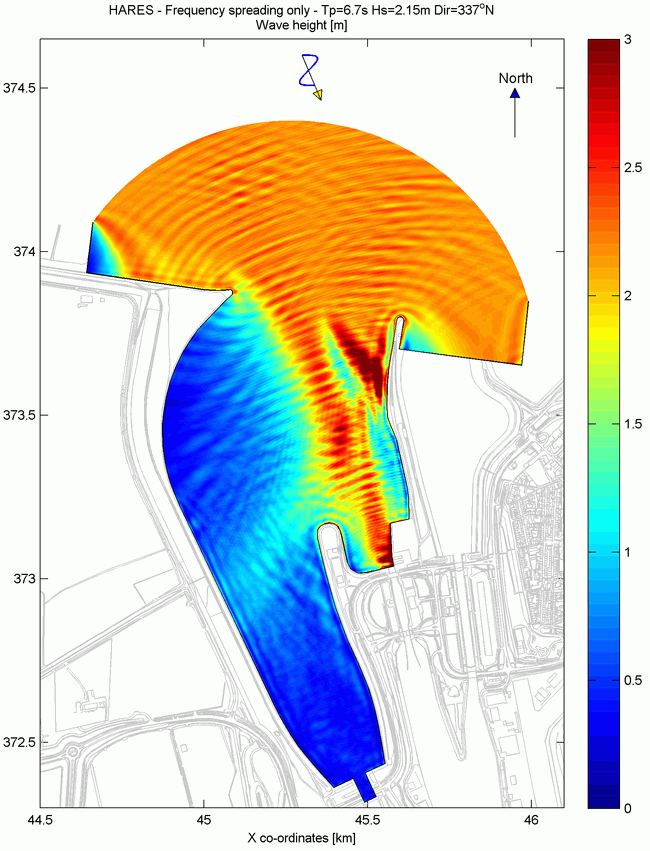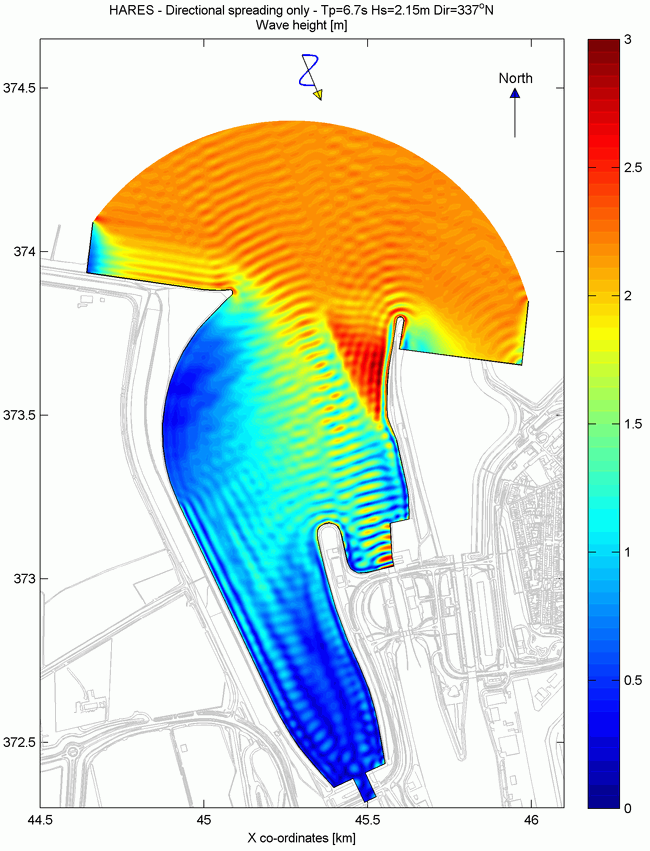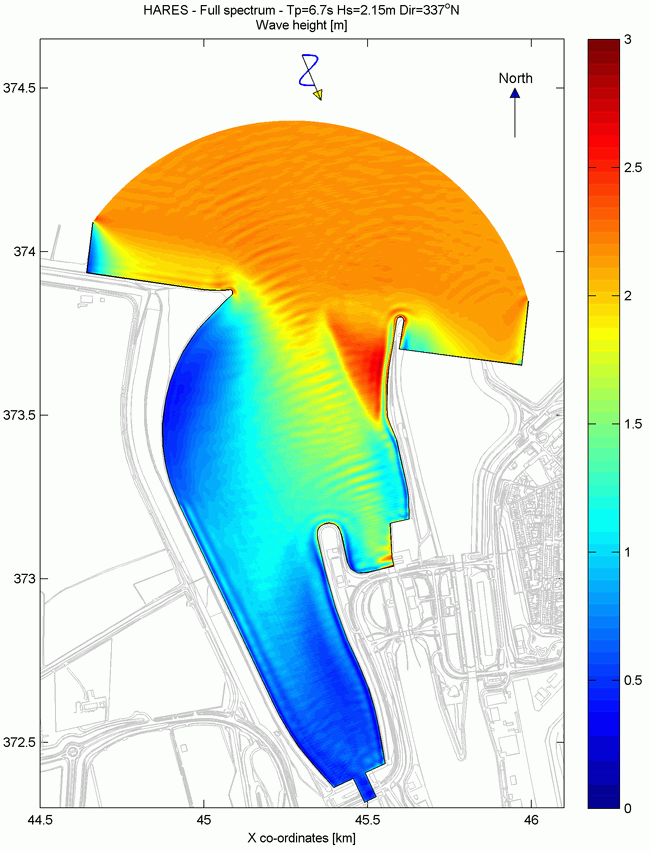# Wave Spectrum Discretisation

For the Port of Terneuzen (the Netherlands) 4 approaches of HARES wave penetration modelling are presented for an extreme wave condition with Tp=6.7s; Hs=2.15m; Dir=337°N; water level=NAP+6m.

For this wave condition a JONSWAP spectrum is assumed with gamma=3.3 and a directional spreading of approximately 25° (m=4.0). The wave spectrum used in the penetration modelling is schematised in 4 different ways, being:

1. propagation of monochromatic wave condition.
2. propagation of wave condition including only frequency spreading.
3. propagation of wave condition including only directional spreading.
4. propagation of wave condition including both frequency and directional spreading (full spectrum).

The discretisation of the imposed wave condition in various frequencies and wave directions is described below.

Monochromatic wave condition
For the monochromatic run only one single wave condition is imposed with only one frequency and one direction.

The figure below depicts the 1D wave spectrum. The wave spectrum is discretised by dividing it into 23 frequencies. The dotted lines in the middle of the coloured bins represent the 23 frequencies that are modelled in HARES, including a line precisely at the peak frequency. For each frequency bin the amount of wave energy is determined. The peak frequency is 0.15Hz, which is equal to a wave period of 6.7 seconds. The last frequency bin contains also all the energy in the tail of the spectrum (f>0.31Hz). The result is a wave spectrum that is discretised into 23 frequency bins where the total wave energy in the bins amounts to 100%.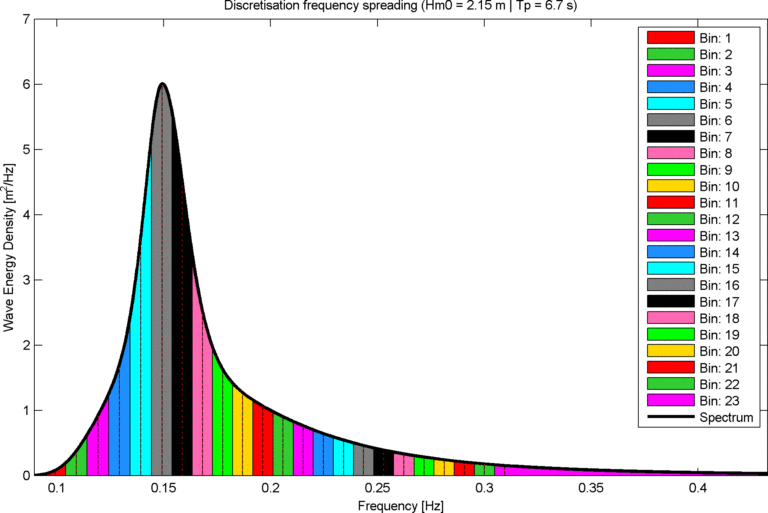The directional spreading is 25° (m=4.0). To include the directional spreading, various HARES simulation runs are carried out for the wave period of 6.7s. The directional spreading is schematised by distributing the wave energy over a main wave direction and wave directions up to 50 degrees at both sides of the main wave direction, with an interval of 5 degrees. The total wave energy is again 100%. The figure below shows the distribution of the wave energy over the 21 wave directions.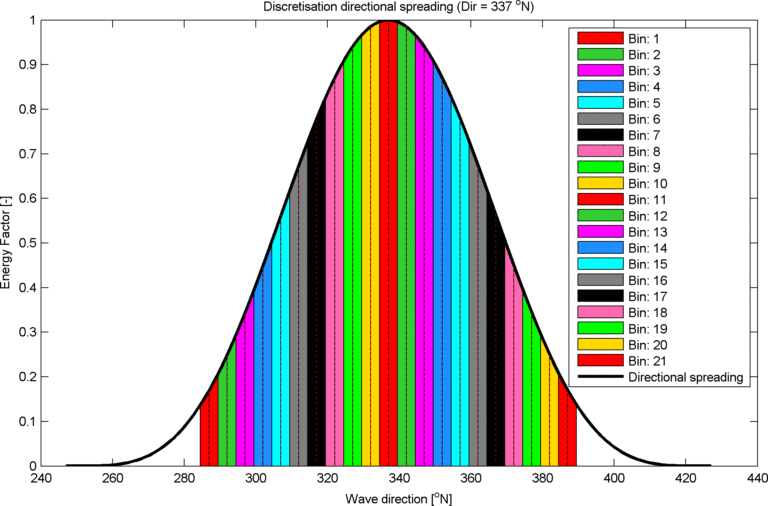Full spectrum
For the fully spectral HARES simulation the 22 frequencies are combined with the 21 wave directions. This HARES simulation will consist of 462 individual runs, each with its own combination of frequency and direction. The total wave energy of all 462 runs amounts to 100%.

HARES has a very fast and efficient spectral treatment of bottom friction and wave breaking based on the entire wave spectrum. To do so, all spectral components are computed within one HARES computation and after each iteration step the entire wave spectrum is reconstructed to calculate the amount of bottom friction and wave breaking. The calculated energy dissipation is then equally distributed among all components.

Results for the Port of Terneuzen
For Port of Terneuzen (satellite image in left panel of figure below) a numerical model is set-up. The computational domain and bathymetry used in the HARES simulations is depicted in the right panel of the figure below. The computational HARES mesh is composed of triangles with a grid size of 1.5m. The total number of triangles is around 1.6 million. During extreme wave events some of the structures in the port might collapse and are therefore not included in the model and/or bathymetry.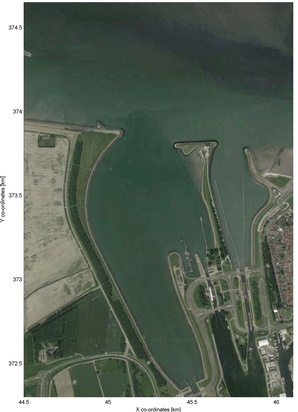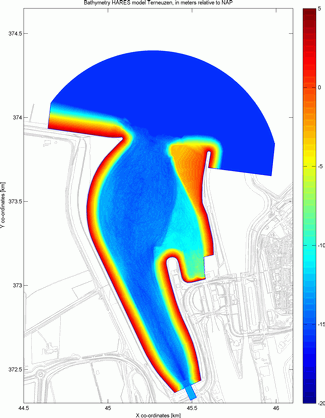The resulting wave patterns of the 4 different schematisations of the wave spectrum are presented in the figures below, with the full spectral schematisation being the most detailed and accurate schematisation approach. These results show that there are significant differences between the fully spectral schematisation approach and the schematisation approaches without directional spreading (i.e. the monochromatic schematisation and the schematisation with only frequency spreading). The difference between the fully spectral schematisation and the schematisation with only direction spreading, on the other hand, are rather small, certainly when the results are averaged over an area in the order of the local wave length. This indicates that direction spreading is an important aspect in the discretisation of the wave spectrum.

The conclusion is that for wave climate studies in relatively open ports the approach with only directional spreading is already satisfactory. In relatively enclosed basins and for detailed analysis of individual waves a full spectral approach is recommended.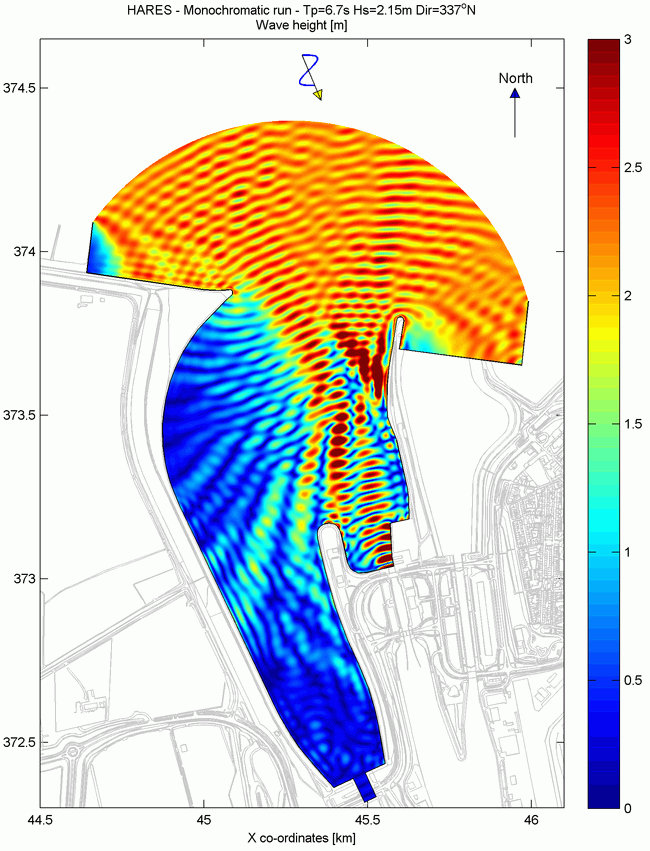Monochromatic run